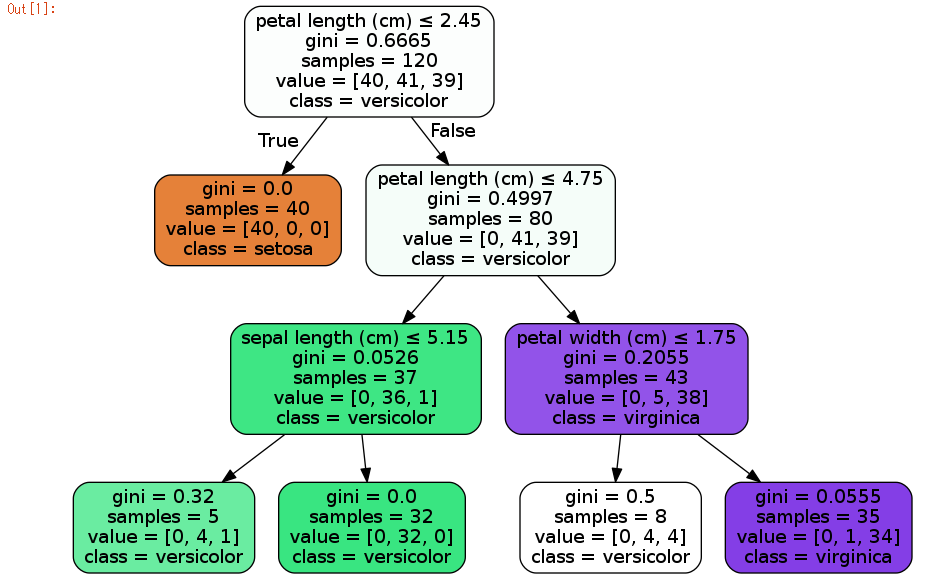Tech Tips

1. Uncategorized
2. 247 view

[Machine Learning]Created docker image including python ML librariesIntroduction

Some time taking much time to install libraries related machine learning and sandbox environment should be messy. So, I created Docker image to make some test with machine learning easy and quick. The image includes main machine learning libraries like tensorflow, chainer and scikit-learn. If you want to check Dockerfile of the image, please see following git repository.

Installed libraries

OS is Ubuntu 16.04 and now following libraries are installed in the image.
• tensorflow 0.12.0
• chainer 1.19.0
• scikit-learn 0.18.1
• gensim 0.13.4
• word2vec 0.9.1
• numpy 1.11.3
• pandas 0.19.2
• jupyter 4.2.1
• matplotlib 1.5.3
• mecab latest
• juman++ 7.01
Of course, dependent libraries are also installed.

How to use

Docker pull and login to container

Just run following commands. In default, jupyter notebook and sudo password is “ml”, so please change it if it’s needed. Here is how to change it. jupyter notebook’s setting file is here /home/ml/.jupyter/jupyter_notebook_config.py in container.

Sample of scikit-learn and mecab/juman++

I try to check I can do a machine learning tasks for sure. At first, I access to the host which run jupyter notebook with browser with 8888 port(ex. http://sample.com:8888). And then, I input “ml” (default password) as login password. I create a notebook to run some python codes. I run following code. The code plot decision tree which classify iris dataset.
import numpy as np
import pandas as pd
from sklearn.cross_validation import ShuffleSplit, train_test_split
from sklearn.tree import DecisionTreeClassifier, export_graphviz
from sklearn.metrics import f1_score, make_scorer, accuracy_score
from sklearn.grid_search import GridSearchCV
from sklearn import datasets
from pydotplus import graph_from_dot_data
from IPython.display import Image

features = iris.data
categories = iris.target

# Cross-Validation setting
X_train, X_test, y_train, y_test = train_test_split(features, categories, test_size=0.2, random_state=42)
cv_sets = ShuffleSplit(X_train.shape, n_iter = 10, test_size = 0.20, random_state = 0)
params = {'max_depth': np.arange(2,11), 'min_samples_leaf': np.array()}

# Learning
def performance_metric(y_true, y_predict):
score = f1_score(y_true, y_predict, average='micro')
return score

classifier = DecisionTreeClassifier()
scoring_fnc = make_scorer(performance_metric)
grid = GridSearchCV(classifier, params, cv=cv_sets, scoring=scoring_fnc)
best_clf = grid.fit(X_train, y_train)

# Plot decision tree
dot_data = export_graphviz(best_clf.best_estimator_, out_file=None,
feature_names=iris.feature_names,
class_names=iris.target_names,
filled=True, rounded=True,
special_characters=True)
graph = graph_from_dot_data(dot_data)
Image(graph.create_png())
Result should be following. So, I can actually use scikit-learn in the container. Next is mecab and juman++. I try to do morphological analysis of a difficult Japanese phrase “すもももももももものうち”.
from MeCab import Tagger
from pyknp import Juman

target_text = u"すもももももももものうち"

m = Tagger("-Owakati")
print("***** Mecab *****")
print(m.parse(target_text))

juman = Juman()
result = juman.analysis(target_text)
print("***** Juman *****")
print(' '.join([mrph.midasi for mrph in result.mrph_list()]))
Result should be following, so I can use python binding of mecab and juman++.

Appendix

I added some samples which includes tflearn and tensorboard. The sample of tflearn and tensorboard is same as following article.